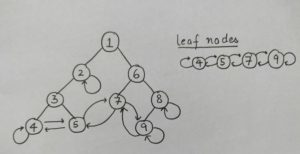Open In App

# OYO Interview Experience – SDE (2019)

Round 1: The first round was hosted on HackerEarth. The test had 2 coding questions and 20 MCQ questions on technical stuff.

There were 4 different types of coding questions, out of which each student had to solve two.

1. Minimum cost to reach the end of a grid. https://www.geeksforgeeks.org/min-cost-path-dp-6/
2. Balloon burst problem. https://www.geeksforgeeks.org/burst-balloon-to-maximize-coins/
3. Replace ‘O’ with ‘X’, if it is surrounded by ‘X’. https://www.geeksforgeeks.org/given-matrix-o-x-replace-o-x-surrounded-x/
4. Scooby has a habit of sleeping in some periods. You are given two arrays: one containing the number of chapters taught for each period and an array containing flags whether scooby sleeps (shown by 0) in that period or not(shown by 1). Your job is to find the period in which you give one scooby-snack to scooby, which is effective for ‘K’ periods such that he covers the maximum chapters.

For example:  chapters covered in each period is [2, 3, 5, 3, 5, 4] and flags for sleep are [1, 1, 0, 1, 0, 0]. That is, scooby is asleep for third, fifth and sixth period. The effectiveness for scooby-snack is 3 periods. The maximum number of chapters covered will be when you give it to scooby in 3rd period.

Number of chapters covered would be : (2 + 3 + 5 + 3 + 5) = 18

In case you give it in 4th period, the chapters would be: (2 + 3 + 3 + 5 + 4) = 17

The MCQs covered technical topics like DBMS, OS, OOPs and Data Structures.

Note: Anyone who solved atleast one of the two coding questions, was qualified for next round.
(22 out of 40 passed)
Round 2: F2F interview

1. You are given a binary tree with some modifications:

a) For any node with exactly one child, the pointer to the absent child points itself. (Say if a node has just left child, then the pointer to the right child points to itself and vice versa).

b) For a leaf node, if there exists a leaf to the right of it (in context to the whole binary tree), then the right child pointer of the leaf node, points to that right leaf, otherwise points to itself.

c) For a leaf node, if there exists a leaf to the left of it (in context to the whole binary tree), then the left child pointer of the leaf node, points to that left leaf, otherwise points to itself.The task was to give the preorder traversal for the binary tree (at the time it was not modified).

For the example given, the traversal should be: 1->2->3->4->5->6->7->8->9

As you can see from the right-hand side figure, the leaves of the Binary Tree make a doubly linked list. Using that property, this question is solved. That is if (current->right)->left = current or (current->left)->right = current,  then the node is a leaf. This would solve the question in O(n) time complexity in O(1) space.

NOTE: The interviewer asked me to code this on paper.

2. Finding the number of perfect squares between two given numbers. https://www.geeksforgeeks.org/find-number-perfect-squares-two-given-numbers/

3. Given a number, find the square root without any in-built function. https://www.geeksforgeeks.org/square-root-of-an-integer/
(9 out of 22 passed)

Round 3: F2F Interview

The interviewer was young and friendly.

The first question was “Tell me about yourself.”

Then started the technical interview:

1. https://www.geeksforgeeks.org/find-the-row-with-maximum-number-1s/
2. Questions on Mutex, Semaphore, Virtual memory vs. RAM
3. A variant of Rotten Oranges question: https://www.geeksforgeeks.org/minimum-time-required-so-that-all-oranges-become-rotten/

NOTE: (The interviewer asked me to code the 3rd question on paper)

I got selected after this round, but few students had to give another round. In the end, 5 out of 9 were selected for the job.

Thanks to GFG archives from where I prepared for the interviews.